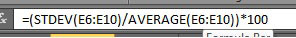# Excel and Relative Standard Deviation %RSD

This guide will detail how to calculated the relative standard deviation (%RSD) using Excel, then walk through a worked example and finally detail the limitations of the calculation. Percentage relative standard deviation is a widely used statistical tool but strangely there is no automated function in any version of Microsoft Excel.

## Relative Standard Deviation in Excel 2003, 2007 & 2010

%RSD is a powerful tool to statistically inspect the variation in sets of data but a specific function is not available in Excel 2003, 2007 or even 2010. To calculate the %RSD in Microsoft Excel a short formula must be used:

= (STDEV(Data Range) / AVERAGE(Data Range))*100

= (STDEV(A1:A11) / AVERAGE(A1:A11))*100The above %RSD example has been entered into the Excel formula bar and will calculate the percent relative standard deviation of the 5 value data set E6 to E11. In the cell where the formula is written a value between 0-100 will be reported. The result is expressed as an percentage, with a low number (<2.5%) indicating a small spread of values and a high value indicating a significant spread of results.

### Why is %RSD So Important?

%RSD (relative standard deviation) is a statistical measurement that describes the spread of data with respect to the mean and the result is expressed as a percentage. The %RSD function is popular with non-statisticians as the interpretation is based on a percent result and not some abstract value. The main use for %RSD is in analytical chemistry and is routinely used to assess the variation of sets of data.

### %RSD Excel Worked Example

The excel %RSD calculation requires two functions; Average and STDEV. The AVERAGE function [=average(population)] calculates the mean of the data set while the STDEV [=STDEV(population)] calculates the standard deviation (SD) of the data. The final stage of the calculation is to express the result as a percent which the *100 does. This following %RSD example is based upon a data set of 5 values. The image on the left displays the full calculation while the right image displays the numerical values.The short version for the left table is to combine the average and SD calculation into one function and this would read:

=(STDEV(population)/(AVERAGE(population))*100

The calculation from the previous example would be expressed as:## Relative Standard Deviation Excel Limitations

Percent relative standard deviation is popular but there are limitations to the statistical method. The main limitation is when the average is very small (<1) and a small variation in the set of data will result in a large result. The data set for %RDS must be based around one set of results, it is not applicable when there are different discrete sets of results. The data set must be larger than 5 for a meaningful result.

Related Pages